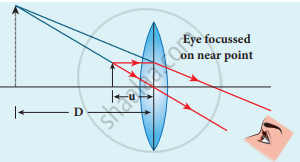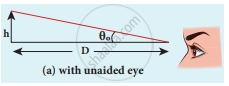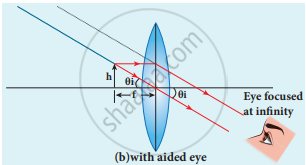Tamil Nadu Board of Secondary EducationHSC Science Class 12th

# Discuss about simple microscope and obtain the equations for magnification for near point focusing and normal focusing. - Physics

Discuss about simple microscope and obtain the equations for magnification for near point focusing and normal focusing.

#### Solution

• A simple microscope is a single magnifying (converging) lens of small focal length.
• The idea is to get an erect, magnified and virtual image of the object. For this the object is placed between F and P on one side of the lens and viewed from other side of the lens.
1. Near point focusing:
The image is formed at a near point, i.e. 25 cm for the normal eye. This distance is also called as least distance D of distinct vision. In this position, the eye feels comfortable but there is little strain on the eye.
2. Normal focusing:
The image is formed at infinity. In this position, the eye is most relaxed to view the image.

Magnification in near point focusing:

1. Object distance u is less than f. The image distance is the near point D. The magnification m is given by the relation,
m = "v"/"u"
2. The magnification can further be written as,
m = 1 - "v"/"f"(using lens equation)
Substituting for ν with sign convention,
v = - D
m = 1 + "D"/"f"
This is the magnification for near-point focusing.Near point focusing

Magnification in normal focusing (angular magnification):

1. The magnification for the image is formed at infinity.
2. The angular magnification is defined as the ratio angle θi, subtended by the image with an aided eye to the angle θ0 subtended by the object with unaided eye.
m = theta_"i"/theta_0Normal focusing

For unaided eye

tan θ0 ≈ θ0 = "h"/"D"

For aided eye

tan θ0 ≈ θ0 = "h"/"f"

The angular magnification is,

m = theta_"i"/theta_0 = ("h"//"f")/("h"//"D")

m = "D"/"f"

This is the magnification for normal focusing.

Concept: Optical Instruments
Is there an error in this question or solution?
Share